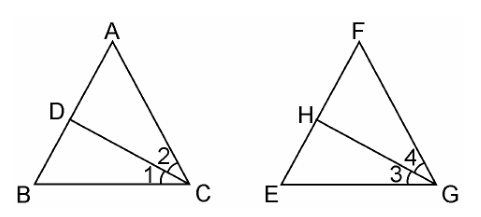# CD and GH are respectively the bisectors of ∠ACB and ∠EGF such that D and H lie on sides AB and FE of ΔABC and ΔEFG respectively. If ΔABC ~ ΔFEG, Show that: (i) CD/GH = AC/FG (ii) ΔDCB ~ ΔHGE (iii) ΔDCA ~ ΔHGF

Given

CD and GH are respectively the bisectors of ∠ACB and ∠EGF such that D and H lie on sides AB and FE of ΔABC and ΔEFG respectively.

To Prove

If ΔABC ~ ΔFEG,

(i) CD/GH = AC/FG
(ii) ΔDCB ~ ΔHGE
(iii) ΔDCA ~ ΔHGF

Proof(i) We have,

ΔABC ~ ΔFEG.

Therefore,

∠A = ∠F, ∠B = ∠E, and ∠ACB = ∠FGE

Now, since,

∠ACB = ∠FGE

∴ ∠ACD = ∠FGH (Angle bisector)

And, ∠DCB = ∠HGE

In ΔACD and ΔFGH,

∠A = ∠F

∠ACD = ∠FGH

By AA similarity criterion, we have,

∴ ΔACD ~ ΔFGH

⇒CD/GH = AC/FG

(ii) In ΔDCB and ΔHGE,

Proved in part (i)

∠DCB = ∠HGE

∠B = ∠E

By AA similarity criterion,

∴ ΔDCB ~ ΔHGE

(iii) In ΔDCA and ΔHGF,

∠ACD = ∠FGH

∠A = ∠F

By AA similarity criterion,

∴ ΔDCA ~ ΔHGF

Hence Proved